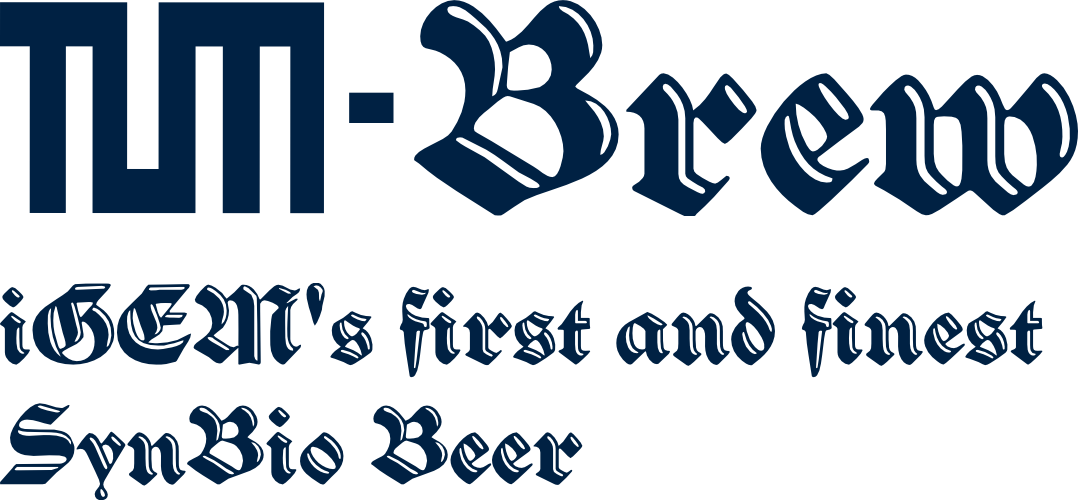# Team:TU Munich/Modeling/Yeast Growth

(diff) ← Older revision | Latest revision (diff) | Newer revision → (diff)# Yeast Growth Model

## Model Selection

As none of our measurement for yeast growth included the stationary phase, fitting a Gompertz curve,

would most likely result in unidentifiabilities as the model is too complex. As the data was normalized to the value at t=0, we fitted it an non-scaled exponential curve.

This means that only one parameter remains, the inverse doubling time, scaled by a factor of ln(2). The normalization ensures that values between measurements are comparable.

## Code

```data1=[1,1.3625,2.875,5.25];

t=[0,80,270,450];

fun = @(k,data) sum(((exp(k(1)*t)-data)/k(2)).^2);

[k, RN] = fminsearch(@(k) fun(k,data1) - length(t)*log(1/(sqrt(2*pi*k(2)))),[0.005 1]);

kc=0.01;
acc=0;
nacc=0;
n=10000;
sample=zeros(n,1);
prob=zeros(n,1);
lh=zeros(n,1);
accepted=zeros(n,1);

kprev = k(1);
s=1;
while (s<n+1)
if(mod(s,500)==0)
(s)/n;
display(['Acceptance Rate: ' num2str(acc/(nacc+acc)*100) '%'])
end
try
% the step is sampled from a multivariate normal distribution and
% scaled with kc
kcur = kprev + kc*mvnrnd(0,0.001);
sample(s,:) = kcur;
% calculate the a-posteriori probability of the new sample
prob(s) = -fun([kcur k(2)],data1);
if s>1
% check whether we reject the sample or not. mind that the
% probability is log-scaled
if log(rand(1)) < +prob(s)-prob(s-1)
% update current sample
kprev = kcur;
% count accepted samples
acc=acc+1;
% save current acceptance rate for post-processing
lh(s)=acc/(nacc+acc)*100;
s=s+1;
else
% count not accepted samples
nacc=nacc+1;
end
else
% we need to do something else for the first sample.
if log(rand(1)) < prob(s)+RN
kprev = kcur;
acc=acc+1;
%waitbar((k-1)/n)
lh(s)=acc/(nacc+acc)*100;
s=s+1;
else
nacc=nacc+1;
end
end
catch ME
disp(ME)
end
end
disp(['Doubling Time: ' num2str(mean(log(2)./sample)) ' STD: ' num2str(std(log(2)./sample))])
```